# Parallel processing inside of a loop operator?

Member Posts: 100Guru
edited June 2019 in Help
I have never seen this before, but there seems to be parallel processing inside of a loop examples operator. I know that in some operators one is able to select parallel execution, but I was always of the opinion it was not possible in Loop Example?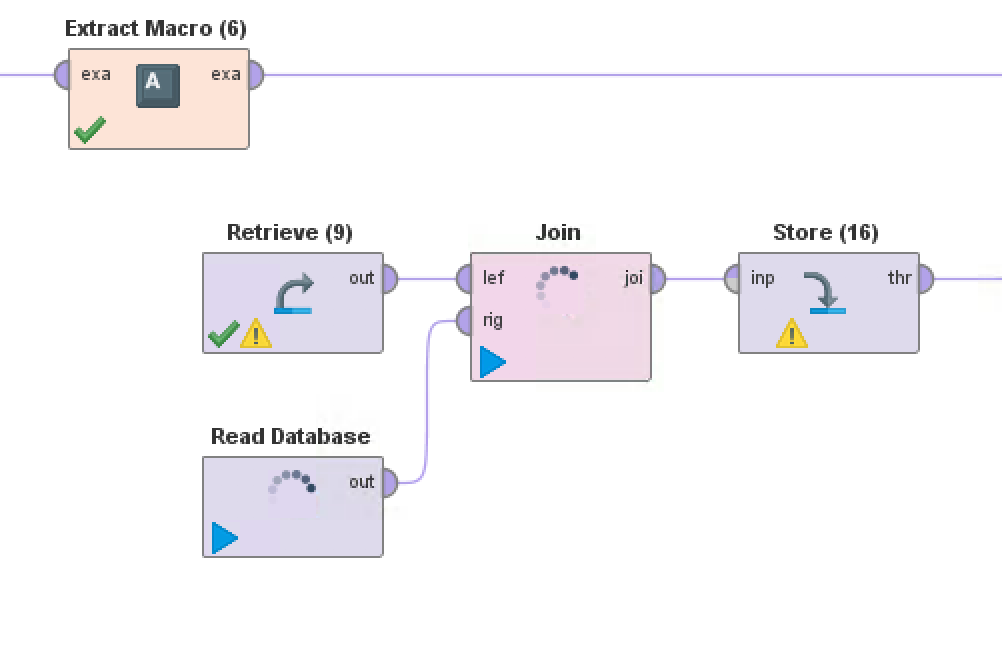Tagged:

• Member Posts: 100Guru
Thanks David, this was something I was unaware of and makes a difference as to how I structure some of the work flows.

• RapidMiner Certified Analyst, Member Posts: 344Unicorn
edited March 2019
Hi @robin

the loop examples operator has shortcommings/bugs, I prefer the normal Loop operator with an Iteration macro, which also has a parallel option.

Regards,
Sebastian

• Administrator, Moderator, Employee, RapidMiner Certified Analyst, Community Manager, Member, University Professor, PM Moderator Posts: 2,959Community Manager
@SGolbert are you referring to any shortcomings/bugs that are not in Prod Feedback / Prod Ideas? Please post if not. It's the only way we know about them.

Thanks.

Scott

• Member Posts: 100Guru
@sgenzer I may be performing this loop incorrectly, but have tried to simulate an issue that I encounter with loop examples. After running through the first example provided, the process does not execute the following examples in the set and says that the parameter does not exist: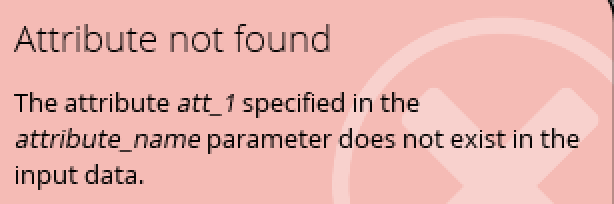```<?xml version="1.0" encoding="UTF-8"?><process version="8.2.000">
<context>
<input/>
<output/>
<macros/>
</context>
<operator activated="true" class="process" compatibility="8.2.000" expanded="true" name="Process">
<process expanded="true">
<operator activated="true" class="generate_data_user_specification" compatibility="8.2.000" expanded="true" height="68" name="Generate Data by User Specification (5)" width="90" x="45" y="238">
<list key="attribute_values">
<parameter key="1" value="(&quot;1&quot;)"/>
<parameter key="2" value="(&quot;2&quot;)"/>
<parameter key="3" value="(&quot;3&quot;)"/>
<parameter key="4" value="(&quot;4&quot;)"/>
<parameter key="5" value="(&quot;5&quot;)"/>
<parameter key="6" value="(&quot;6&quot;)"/>
<parameter key="7" value="(&quot;7&quot;)"/>
<parameter key="8" value="(&quot;8&quot;)"/>
<parameter key="9" value="(&quot;9&quot;)"/>
<parameter key="a" value="(&quot;a&quot;)"/>
<parameter key="b" value="(&quot;b&quot;)"/>
<parameter key="c" value="(&quot;c&quot;)"/>
<parameter key="d" value="(&quot;d&quot;)"/>
<parameter key="e" value="(&quot;e&quot;)"/>
<parameter key="f" value="(&quot;f&quot;)"/>
</list>
<description align="center" color="transparent" colored="false" width="126">Generate the prefixes that will be used in the loop operator</description>
</operator>
<operator activated="true" class="transpose" compatibility="8.2.000" expanded="true" height="82" name="Transpose (5)" width="90" x="179" y="238"/>
<operator activated="true" class="loop_examples" compatibility="8.2.000" expanded="true" height="82" name="Loop Examples (5)" width="90" x="313" y="238">
<process expanded="true">
<operator activated="true" class="extract_macro" compatibility="8.2.000" expanded="true" height="68" name="Extract Macro (7)" width="90" x="112" y="34">
<parameter key="macro" value="prefix"/>
<parameter key="macro_type" value="data_value"/>
<parameter key="attribute_name" value="att_1"/>
<parameter key="example_index" value="%{example}"/>
</operator>
<operator activated="true" class="generate_data_user_specification" compatibility="8.2.000" expanded="true" height="68" name="Generate Data by User Specification" width="90" x="112" y="289">
<list key="attribute_values">
<parameter key="2" value="&quot;a&quot;"/>
<parameter key="2" value="&quot;b&quot;"/>
<parameter key="2" value="&quot;c&quot;"/>
</list>
</operator>
<operator activated="true" class="filter_examples" compatibility="8.2.000" expanded="true" height="103" name="Filter Examples" width="90" x="246" y="289">
<list key="filters_list">
<parameter key="filters_entry_key" value="2.does_not_contain.%{prefix}"/>
</list>
<parameter key="filters_logic_and" value="false"/>
</operator>
<operator activated="true" class="generate_data_user_specification" compatibility="8.2.000" expanded="true" height="68" name="Generate Data by User Specification (2)" width="90" x="112" y="136">
<list key="attribute_values">
<parameter key="1" value="&quot;a&quot;"/>
<parameter key="1" value="&quot;b&quot;"/>
<parameter key="1" value="&quot;c&quot;"/>
<parameter key="1" value="&quot;d&quot;"/>
<parameter key="1" value="&quot;e&quot;"/>
</list>
</operator>
<operator activated="true" class="filter_examples" compatibility="8.2.000" expanded="true" height="103" name="Filter Examples (2)" width="90" x="246" y="136">
<list key="filters_list">
<parameter key="filters_entry_key" value="1.does_not_contain.%{prefix}"/>
</list>
<parameter key="filters_logic_and" value="false"/>
</operator>
<operator activated="true" class="concurrency:join" compatibility="8.2.000" expanded="true" height="82" name="Join (31)" width="90" x="447" y="136">
<parameter key="join_type" value="outer"/>
<parameter key="use_id_attribute_as_key" value="false"/>
<list key="key_attributes">
<parameter key="1" value="2"/>
</list>
<parameter key="keep_both_join_attributes" value="true"/>
</operator>
<operator activated="true" class="generate_data_user_specification" compatibility="8.2.000" expanded="true" height="68" name="Generate Data by User Specification (3)" width="90" x="112" y="748">
<list key="attribute_values">
<parameter key="1" value="&quot;e&quot;"/>
<parameter key="1" value="&quot;f&quot;"/>
<parameter key="1" value="&quot;g&quot;"/>
</list>
</operator>
<operator activated="true" class="filter_examples" compatibility="8.2.000" expanded="true" height="103" name="Filter Examples (3)" width="90" x="246" y="748">
<list key="filters_list">
<parameter key="filters_entry_key" value="1.does_not_contain.%{prefix}"/>
</list>
<parameter key="filters_logic_and" value="false"/>
</operator>
<operator activated="true" class="remember" compatibility="8.2.000" expanded="true" height="68" name="Remember" width="90" x="581" y="136">
<parameter key="name" value="data"/>
</operator>
<operator activated="true" class="free_memory" compatibility="8.2.000" expanded="true" height="82" name="Free Memory (32)" width="90" x="715" y="136"/>
<operator activated="true" class="recall" compatibility="8.2.000" expanded="true" height="68" name="Recall" width="90" x="112" y="595">
<parameter key="name" value="data"/>
</operator>
<operator activated="true" class="filter_examples" compatibility="8.2.000" expanded="true" height="103" name="Filter Examples (4)" width="90" x="246" y="595">
<list key="filters_list">
<parameter key="filters_entry_key" value="1.does_not_contain.%{prefix}"/>
</list>
<parameter key="filters_logic_and" value="false"/>
</operator>
<operator activated="true" class="concurrency:join" compatibility="8.2.000" expanded="true" height="82" name="Join (2)" width="90" x="447" y="595">
<parameter key="join_type" value="left"/>
<parameter key="use_id_attribute_as_key" value="false"/>
<list key="key_attributes">
<parameter key="1" value="1"/>
</list>
</operator>
<operator activated="true" class="store" compatibility="8.2.000" expanded="true" height="68" name="Store (2)" width="90" x="581" y="595">
<parameter key="repository_entry" value="//Local Repository/data/AOL/AOL database full cvm"/>
</operator>
<operator activated="true" class="free_memory" compatibility="8.2.000" expanded="true" height="82" name="Free Memory (2)" width="90" x="715" y="595"/>
<connect from_port="example set" to_op="Extract Macro (7)" to_port="example set"/>
<connect from_op="Generate Data by User Specification" from_port="output" to_op="Filter Examples" to_port="example set input"/>
<connect from_op="Filter Examples" from_port="example set output" to_op="Join (31)" to_port="right"/>
<connect from_op="Generate Data by User Specification (2)" from_port="output" to_op="Filter Examples (2)" to_port="example set input"/>
<connect from_op="Filter Examples (2)" from_port="example set output" to_op="Join (31)" to_port="left"/>
<connect from_op="Join (31)" from_port="join" to_op="Remember" to_port="store"/>
<connect from_op="Generate Data by User Specification (3)" from_port="output" to_op="Filter Examples (3)" to_port="example set input"/>
<connect from_op="Filter Examples (3)" from_port="example set output" to_op="Join (2)" to_port="right"/>
<connect from_op="Remember" from_port="stored" to_op="Free Memory (32)" to_port="through 1"/>
<connect from_op="Recall" from_port="result" to_op="Filter Examples (4)" to_port="example set input"/>
<connect from_op="Filter Examples (4)" from_port="example set output" to_op="Join (2)" to_port="left"/>
<connect from_op="Join (2)" from_port="join" to_op="Store (2)" to_port="input"/>
<connect from_op="Store (2)" from_port="through" to_op="Free Memory (2)" to_port="through 1"/>
<connect from_op="Free Memory (2)" from_port="through 1" to_port="example set"/>
<portSpacing port="source_example set" spacing="0"/>
<portSpacing port="sink_example set" spacing="0"/>
<portSpacing port="sink_output 1" spacing="0"/>
</process>
<description align="center" color="transparent" colored="false" width="126"/>
</operator>
<connect from_op="Generate Data by User Specification (5)" from_port="output" to_op="Transpose (5)" to_port="example set input"/>
<connect from_op="Transpose (5)" from_port="example set output" to_op="Loop Examples (5)" to_port="example set"/>
<connect from_op="Loop Examples (5)" from_port="example set" to_port="result 1"/>
<portSpacing port="source_input 1" spacing="0"/>
<portSpacing port="sink_result 1" spacing="0"/>
<portSpacing port="sink_result 2" spacing="0"/>
</process>
</operator>
</process>```

• Administrator, Moderator, Employee, RapidMiner Certified Analyst, Community Manager, Member, University Professor, PM Moderator Posts: 2,959Community Manager
edited March 2019
aha yup. You need to connect to the 'out' port inside the Loop Examples operator - not the 'exa' port: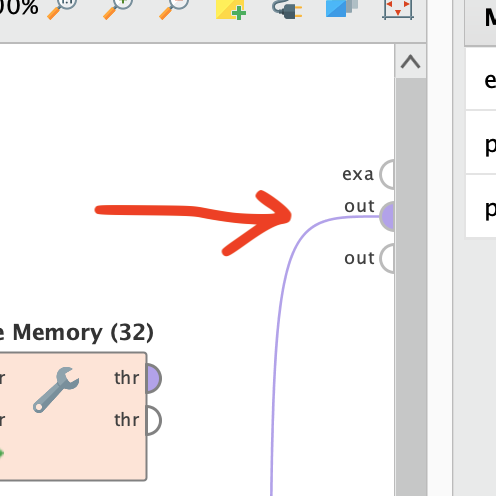It's pretty sneaky - the 'exa' port will RESEND the data back to the input 'exa' port of Loop Examples for each iteration; the 'out' port will not. So after your first iteration the way you had it, the data coming into Extract Macro (7) was the data that went out of Join (2) after the previous iteration.

Clear as mud? That's not a bug - that's just the way Loop Examples works.

Scott

[EDIT FWIW the help panel does try to explain this...]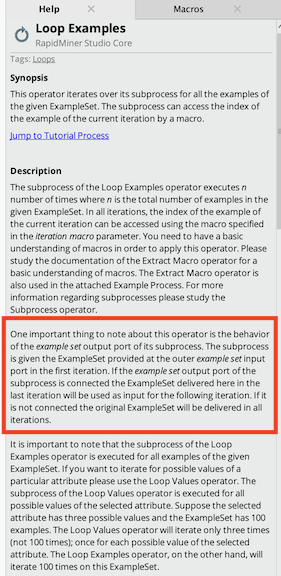• Member Posts: 100Guru

One important thing to note about this operator is the behavior of the example setoutput port of its subprocess. The subprocess is given the ExampleSet provided at the outer example setinput port in the first iteration. If the example setoutput port of the subprocess is connected the ExampleSet delivered here in the last iteration will be used as input for the following iteration. If it is not connected the original ExampleSet will be delivered in all iterations.anw

Cause, I did not pick up anywhere that this is how the operator works. So yip, pretty muddy.

• RapidMiner Certified Analyst, Member Posts: 344Unicorn

as you said that's probably not a bug, but at least to me the operator is so unintuitive to the point of being a big productivity issue. Provided that it has been buggy before, I've given up on it.

My desired behaviour would be an operator that throws a single row into the subprocess, or at least simulates this behaviour. I currently do this with a Loop operator and Filter Examples Range operator inside the subprocess.

Regards,
Sebastian

• Administrator, Moderator, Employee, RapidMiner Certified Analyst, Community Manager, Member, University Professor, PM Moderator Posts: 2,959Community Manager
@SGolbert perfectly fair opinion. For me I'm totally used to the way Loop Examples and Loop Values work...but I work with them practically every day. Feel free to post a new discussion along these lines and tag it Feature Request.

Scott

• Member Posts: 100Guru
Pronouns are you enemy in help files, try not to use them. When you say 'it', which 'it' are you referring to. I read that help file numerous times and still did not understand what was being said. I had to re-write it to understand what was being communicated:

One important note on the behaviour of the example set output port for Loop Examples, the first iteration of Loop Examples uses the ExampleSet provided at the outer example set input port, for the next iteration if the output from the process is connected to the example set output port and not to the output port then the ExampleSet delivered to the example set port will be used for this iteration. Connecting the output to the output port means the process will then use the input port ExampleSet in the next iteration. If the output is not connected to either of the ports then the input port ExampleSet will be delivered in all iterations.
• Administrator, Moderator, Employee, RapidMiner Certified Analyst, RapidMiner Certified Expert, Community Manager, RMResearcher, Member, University Professor Posts: 1,751RM Founder
Awesome, thanks for your help on this.  Scott, I have forwarded this to our tech docs team.
Best,
Ingo
• Employee, Member Posts: 2RM Team Member
• Member Posts: 3Contributor I
Hi @David_A

Can you provide the list of parallelized Operator?
Can we
run spark in parallel mode in standard Loop Values?

I have tried using standard “Loop Values” with enable parallel execution by Inside the loop values operator, using  Radoop nest with SparkRM  as shown below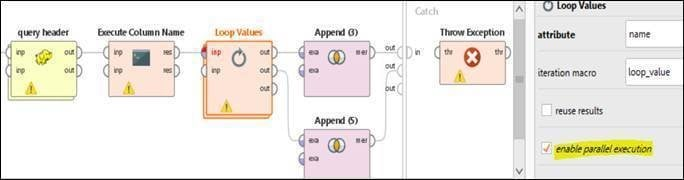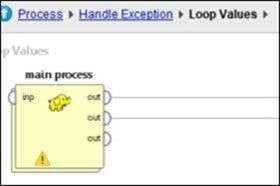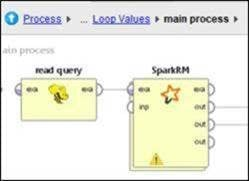I ran this workflow on AI hub server, but I got error. If I use the same flow without enable parallel execution on Loop values operator. The flow works smoothly without error but it is quite slow.

Any suggestion?
• Administrator, Moderator, Employee, RapidMiner Certified Analyst, RapidMiner Certified Expert, University Professor Posts: 3,453RM Data Scientist
Hi,
please consult your customer success manager, so that we can look at the errors together.

What you do here is send tons of concurrent jobs to your Hadoop, which in turn sends parallel jobs to spark. So this is at least 3 levels of parallelization. One needs to look carefully and not from a 10.000 foot view to understand the error.

Best,
Martin
- Sr. Director Data Solutions, Altair RapidMiner -
Dortmund, Germany